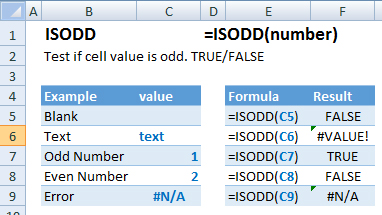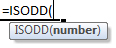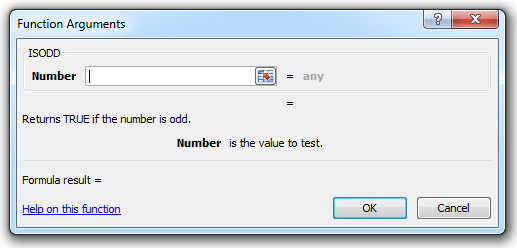### ISODD Function Examples – Excel, VBA, & Google Sheets

This Excel Tutorial demonstrates how to use the Excel ISODD Function in Excel to test if a cell value is odd, with formula examples.

## ISODD Function Description:

The ISODD Function Test if cell value is odd. Returns TRUE or FALSE.

## Formula Examples:Example Formula Result
Blank =ISODD(C5) False
Text =ISODD(C6) #VALUE!
Odd Number =ISODD(C7) True
Even Number =ISODD(C8) False
Error =ISODD(C9) #N/A
AutoMacro - VBA Code Generator

## Syntax and Arguments:

The Syntax for the ISODD Formula is:

`=ISODD(number)`

### Function Arguments ( Inputs ):

value – The test value

## Additional Notes

Use the ISODD Function to test if cell value is odd. true/false

## ISODD Examples in VBAYou can also use the ISODD function in VBA. Type:
`Application.Worksheetfunction.Isodd(number)`
For the function argument (number), you can either enter them directly into the function, or define variables to use instead.

Return to the List of all Functions in Excel

## How to use the ISODD Function in Excel:

To use the AND Excel Worksheet Function, type the following into a cell:
`=AND(`
After entering it in the cell, notice how the AND formula inputs appear below the cell:You will need to enter these inputs into the function. The function inputs are covered in more detail in the next section. However, if you ever need more help with the function, after typing “=ISODD(” into a cell, without leaving the cell, use the shortcut CTRL + A (A for Arguments) to open the “Insert Function Dialog Box” for detailed instructions:For more information about the ISODD Formula visit the
Microsoft Website.

## Excel Practice Worksheet

Practice Excel functions and formulas with our 100% free practice worksheets!

• Automatically Graded Exercises
• Learn Excel, Inside Excel!